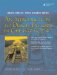# Enumerations

In Chapter 2 we discussed at some length how we can add new types to the C++ language by defining classes. Another way to add new types to C++ deserves some more discussion.

The keyword enum is used for assigning integral values to C++ identifiers. For example, when designing data structures that perform bitwise operations, it is very convenient to give names to the various bitmasks. The main purpose for an enum is to make the code more readable and, hence, easier to maintain. For example,

```enum {UNKNOWN, JAN, FEB, MAR };
```

defines three constant identifiers, numbered in ascending order, starting at 0. It is equivalent to

```enum {UNKNOWN=0, JAN=1, FEB=2, MAR=3};
```

The identifiers, JAN, FEB, and MAR are called enumerators.

They can be defined and initialized to arbitrary integer values.

```enum Ages {manny = 10, moe, jack = 23,
scooter = jack + 10};
```

If the first enumerator, manny, had not been initialized, it would have been given the value 0. Since we initialized manny to 10 and we did not assign a value to moe, the value of moe is 11. The values of enumerators need not be distinct.

When an enum has a tag name, then a new type is defined. For example,

```enum Winter {JAN=1, FEB, MAR, MARCH = MAR };
```

The name Winter is called a tag name. Now, we can declare variables of type Winter.

```Winter m = JAN;
int i = JAN; // OK - enum can be implicitly converted to int
m = i; // ERROR! An explicit cast is required.
m = static_cast(i); // OK
i = m; // OK
m = 4; // ERROR
```

The tag name and the enumerators must be distinct identifiers within their scope.

Enumerations can be implicitly converted to ordinary integer types, but the reverse is not possible without an explicit cast. Example 19.1 demonstrates the use of enum and also shows how the compiler symbols look when they are printed out.

Example 19.1. src/enums/enumtst.cpp

 ```#include using namespace std; int main(int, char** ) { enum Signal { off, on } sig; <-- 1 sig = on; enum Answer { no, yes, maybe = -1 }; <-- 2 Answer ans = no; <-- 3 enum { lazy, hazy, crazy } why; <-- 4 int i, j = on; <-- 5 sig = off; i = ans; // ans = sig; <-- 6 ans = static_cast(sig); <-- 7 ans = (sig ? no : yes); why = hazy; cout << "sig, ans, i, j, why " << sig << ans << i << j << why << endl; return 0; } Output: OOP> gpp enumtest.cc a, b, i, j, why 01011 OOP> ```   (1)a new type, two new enum identifiers, and a variable definition all in one line (2)just the type/enum definitions (3)an instance of an enum (4)a typeless enum variable (5)An enum can always convert to int. (6)Conversions between enum types cannot be done implicitly. (7)Conversion is okay with a cast.An Introduction to Design Patterns in C++ with Qt 4
ISBN: 0131879057
EAN: 2147483647
Year: 2004
Pages: 268